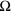# Quantities and Units

## Quantities

The table shows electrical quantities which are used in electronics.

The relationship between quantities can be written using words or symbols (letters), but symbols are normally used because they are much shorter; for example V is used for voltage, I for current and R for resistance.

For example, this is a word equation:

 voltage = current × resistance

And the same equation using symbols:

 V = I × R

To prevent confusion we normally use the same symbol (letter) for each quantity and these symbols are shown in the second column of the table.

 Quantity UsualSymbol Unit UnitSymbol Voltage V volt V Current I amp* A Charge Q coulomb C Resistance R ohmCapacitance C farad F Inductance L henry H Reactance X ohmImpedance Z ohmPower P watt W Energy E joule J Time t second s Frequency f hertz Hz * strictly the unit is ampere, but this is almost always shortened to amp.

## Units

The table above shows the unit (and unit symbol) which is used to measure each quantity. For example: charge is measured in coulombs and the symbol for a coulomb is C.

Some of the units have a convenient size for electronics, but most are either too large or too small to be used directly so they are used with prefixes.

The prefixes shown make the unit larger or smaller by the value shown.

Some examples:

• 25mA = 25 × 10-3A = 25 × 0.001A = 0.025A
• 47µF = 47 × 10-6 F = 47 × 0.000001F = 0.000047F
• 270k= 270 × 103= 270 × 1000= 270000Prefix Value milli m 10-3 = 0.001 micro µ 10-6 = 0.000 001 nano n 10-9 = 0.000 000 001 pico p 10-12 = 0.000 000 000 001 kilo k 103 = 1000 mega M 106 = 1000 000 giga G 109 = 1000 000 000 tera T 1012 = 1000 000 000 000

### Why not change the units to be better sizes?

It might seem a good idea to make the farad (F) much smaller to avoid having to use µF, nF and pF, but if we did this most of the equations in electronics would have to have factors of 1000000 or more included as well as the quantities. Overall it is much better to have the units with their present sizes which are defined logically from the equations.

In fact if you use an equation frequently you can use special sets of prefixed units which are more convenient.

For example: Ohm's Law, V = I × R

The standard units are volt (V), amp (A) and ohm (), but you could use volt (V), milliamp (mA) and kilo-ohm (k) if you prefer.

Take care though, you must never mix sets of units: using V, A and kin Ohm's Law would give you wrong values.